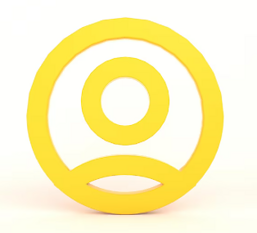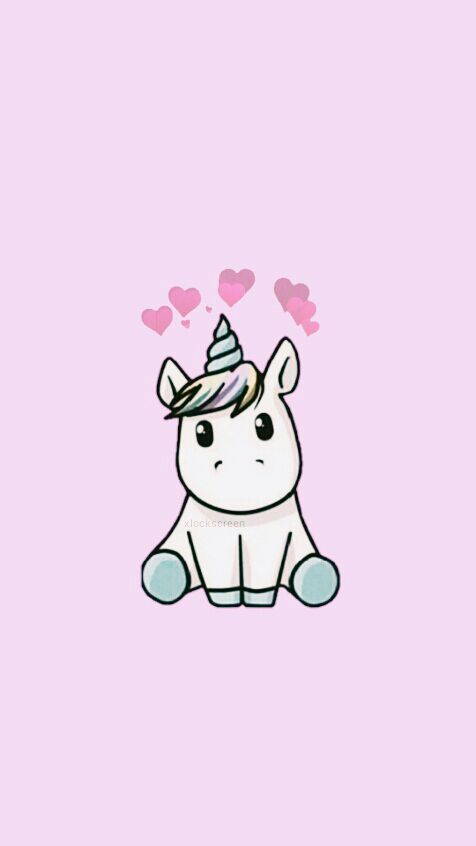(5/5)

### Solve as initial value problem. The number of bacteria is a dish doubles every 10 hours. The number of bacteria starts off at 10.

INSTRUCTIONS TO CANDIDATES

Place code for initial value problems worksheet here. You only need to place code for the problems in BOLD.

1. Solve as initial value problem. The number of bacteria is a dish doubles every 10 hours. The number of bacteria starts off at 10.

1. How many bacteria are in the dish after 70 hours. Set this up as an initial value problem using c++. (I know you can do it with your calculator.)

2. How how long until the number of bacteria exceed 100,000,000?

1. Solve as initial value problem. The force on a 1000kg car is described according to F = 300t^2 - 2000t. Determine how far the car goes between time = 2 sec and time  = 5 seconds. Hint: start the time at 2 seconds and the position at 0 m.

1. Solve as initial value problem. A car starts at a stop sign with an acceleration of 5 m/s^2. How far does the car go after 10 seconds? If you are in physics class, check your work with your physics knowledge. Print the car’s velocity as a function of time. Redirect the output to a file. Create a graph of the car’s velocity as a function of time during these 10 seconds.

(5/5)

## Related Questions

##### Introgramming & Unix Fall 2018, CRN 44882, Oakland University Homework Assignment 6 - Using Arrays and Functions in C

DescriptionIn this final assignment, the students will demonstrate their ability to apply two ma

##### The standard path finding involves finding the (shortest) path from an origin to a destination, typically on a map. This is an

Path finding involves finding a path from A to B. Typically we want the path to have certain properties,such as being the shortest or to avoid going t

##### Develop a program to emulate a purchase transaction at a retail store. This program will have two classes, a LineItem class and a Transaction class. The LineItem class will represent an individual

Develop a program to emulate a purchase transaction at a retail store. Thisprogram will have two classes, a LineItem class and a Transaction class. Th

##### SeaPort Project series For this set of projects for the course, we wish to simulate some of the aspects of a number of Sea Ports. Here are the classes and their instance variables we wish to define:

1 Project 1 Introduction - the SeaPort Project series For this set of projects for the course, we wish to simulate some of the aspects of a number of

##### Project 2 Introduction - the SeaPort Project series For this set of projects for the course, we wish to simulate some of the aspects of a number of Sea Ports. Here are the classes and their instance variables we wish to define:

1 Project 2 Introduction - the SeaPort Project series For this set of projects for the course, we wish to simulate some of the aspects of a number of

### Ask This Assignment To Be Done By Our ExpertsGet A+ Grade Solution Guaranteed##### joyComputer science
(4/5)